# Determination of the threshold by the D-max method: logic and math.

Share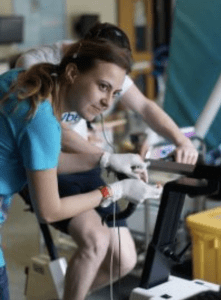The D-max point on the curve is mathematically the point at which the growth rate of the parameter is equal to its average growth rate in the test.

Written by Peter Joffe

In this article, I’m going to explain the simple mathematical logic of the D-max method for determining thresholds. I think it’s always good to understand the essence of the methods we use in science. Despite the fact that this method is widely used in research, I have never seen an explanation of its logic*. Perhaps I didn’t search hard enough. Additionally, based on this logic, I have created an online tool for automated calculation of D-max point.

### Thresholds.

Exercise thresholds are perhaps one of the most popular and widely discussed topics in sports physiology. And this is not surprising. Our intuition and everyday experience perceive the threshold as a kind of cliff. You can be safe in front of it even if you are close to the edge, and you will die immediately if you step even a few centimetres over it.
Thus it would be good to know where this edge exactly is and build an exercise programme around it.

In fact, this intuitive understanding is not entirely correct. Physiological changes in the body during exercise accumulate gradually, and usually there is no sharp breakdown at some distinct moment. The process is more like an exponential curve that accelerates when exercise intensity increases, rather than sudden fall into an abyss.

So, the threshold is some point on this curve, which we have chosen for some reason.
What are the reasons and criteria?

The most common and simplified explanation for the threshold is “anaerobic“.
The body cannot fully meet its energy needs through aerobic production and begins to use the anaerobic pathway as well. This leads to the depletion of the limited “anaerobic reserve” or, more likely, to the rapid accumulation of metabolites and the inevitable cessation of work.

Well, as I said, this is a simplification of the process of developing fatigue during exercise. Nevertheless it can be taken for practical purposes.

### How can we find threshold?

Basically there are two methods based on blood and breath analysis.
Of course, everyone has heard of lactic acid. Accumulation of it in the blood for a century was considered the cause of fatigue. So even the most common name for the threshold is “lactate threshold.” Now we know it’s not that simple (how many times will I use this phrase?). Lactate metabolism during exercise is a rather complex and exciting topic (see article).
However, the blood lactate (BL)  curve during the incremental test definitely reflects the  exercise difficulty.

The same is true for breathing. And the second most used name for threshold definition is “ventilatory”.
Again, the connection between breathing and fatigue is quite complex (see article) and will not be discussed here. For the purposes of this article, it is simply important to know that the breath during the incremental test also follows an exponential curve.

It is worth noting that the curves of BL and respiration during exercise are different. What I mean is that while both reflect fatigue and are correlated, they are not causally related, so they can have different shapes and define threshold differently. Lactate and respiratory thresholds, expressed by intensity, usually do not coincide!
Moreover, even within the same domain (breath or blood), the curve can be different depending on the design of the test.

Therefore, in my opinion, there is no “absolute threshold” that can be expressed in universal speed or intensity.
The threshold is rather a kind of borderline state of the body, when a relatively small increase in intensity leads to a relatively rapid development of fatigue.
This can occur at different speeds, loads and intensities depending on the conditions of the exercise and the form of the athlete.

Thus, in my opinion, the usefulness of using the threshold intensity for exercise prescription  is limited. Especially for experienced athletics coaches who have mastered prescribing exercises with speed at different distances for reference.

However, threshold tests are certainly useful for tracking progress. Fitter athletes show a shift to the right of the BL or breathing curve compared to less trained counterparts. This means that the development of fatigue starts to accelerate at higher intensity (later).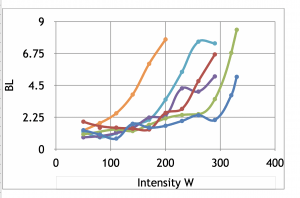Stepwise incremental lactate test for six participants. Lactate curves reflect level of fitness.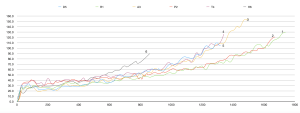Ventilation curves for six participants during incremental stepwise test. Right-shift reflects level of fitness.

### Going to the point.

So let’s move to threshold determination part.
Ok, we conducted an incremental test and we have a curve. On X-axis — intensity/speed/workload, on Y-axis — parameter (BL, respiration, etc.)
How can we define some point on the curve as a threshold?

One of the most popular methods for that is, so called, D-max method.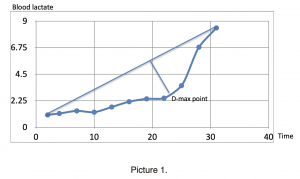D-max is the point on the curve (for example, blood lactate or respiration) that is furthest from the line between the start and end of the curve (see picture 1).
Usually this point is selected visually/intuitively by an experienced practitioner.
Well, that’s pretty subjective. Can we develop some algorithm that will solve this task?

### Math.

Let’s try to implement math. As an example, I describe mathematical logic behind the D-max threshold determination from real-life BL testing (picture1, table 1).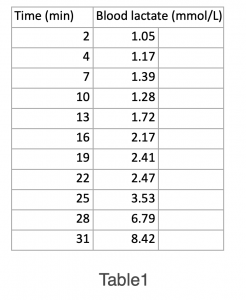On the graph: X-axis —time. On Y-axis —BL.
We can always find out from the test design how the increment was made. In this example, the ergometer test began with a 4-minute warm-up at 50 watts. After that, the increment was 30 watts every 3 minutes, so we can easily convert time to intensity.

First, let’s calculate the fit-line for this blood lactate  plot and the polynomial function for this line (picture 2). Typically, a 3-rd order polynomial function gives a good estimate of the lactate and respiration plots. In our example, the coefficient of determination is:R2=0.9738
Polinomial function: y= 0.0008x3 – 0.0256x2 + 0.2852x + 0.4542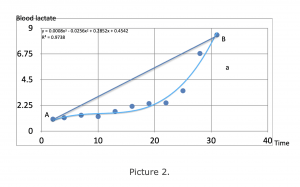Next we need to find a linear equation for the line AB which connects starting and ending points on the curve. In our case it is:
y=0.2541x + 0.5417.

Actually, we need to know only a slope of the line, which is a coefficient in its equation. It can be found simply by:
Y(n)-Y(1)/X(n)-X(1)
Here it is: (8.42-1.05)/(31-2)=0.2541

Basically it is increase of parameter divided by increase of
intensity/time. That is average speed of increase.
In this example, BL increase is 0.2541 mmol/l*min.

After that, according to D-max method we need to find point on curve (in this case on fit-line) which is furthest from the line AB.
This point will be at the place where the line “a”, tangent to the curve, is parallel to the line AB, according to the tangent-radius theorem (picture 3).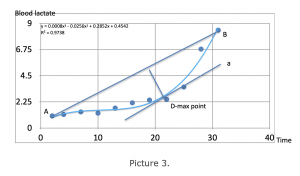What does parallelism between two lines mean mathematically?
This means that the slopes of the lines are the same. Therefore, they go up at the same rate. Consequently, the derivative of the polynomial function at the point D-max –  the speed of the function, coincides with the slope of the line AB  (0.2541 mmol / l * min). This slope represents the average rate of increase in blood lactate during our test.

Thus we can make an important conclusion:

Point D-max on the BL curve mathematically represents point where speed of increase of BL is equal to the average speed of BL rise in the test.

Now we can build simple mathematical algorithm which gives us D-max point:
1. Build a fit-line for the graph (3-d order polynomial) with coefficients a b c and d
2. Find the average speed of increase of parameter (slope for the line between start and end of the test). It can be found:
Y(n)-Y(1)/X(n)-X(1)
3. Find derivative for 3-d order polynomial. It is:

3ax2 + 2bx + c

3ax2 + 2bx + c=Y(n)-Y(1)/X(n)-X(1).

In our example polynomial coefficients are:
a:0.0008 b: -0.0256 c: 0.2852

Therefore:

0.0024x2-0.0512x+0.2852-0.2541=0

5. Take only the largest root.

D-max= 20.7 min

### Implementation.

The D-max method is vulnerable to starting point selection. That affects the calculation of the average speed. Therefore, this procedure should be standard. In my opinion, it is better to take data after warming up. At this point, the measured parameter should already stabilise and begin to behave more logically.

When it comes to respiration data, it’s best to take averages rather than raw numbers. For example, it could be a moving average.

Portable devices provide good opportunity for threshold’s testing without usage of laboratories.
For example, BL meters are available for years. New field devices for measuring respiration become popular also. They are ventilation vests or belts. They measure chest movement for extrapolation of ventilation.

However, there is a problem: ventilation volume is the product of tidal volume (the amount of air per breath) and the respiratory rate. Both change during exercise. Measuring the respiratory rate, as far as I understand, is relatively reliable: you need to measure the movements of the chest. Reliably calculating tidal volume (without actually measuring it) is much more difficult. You need at least a calibration.

Breath frequency itself can be used as an indication of the difficulty of the exercise. It correlates with other parameters used to determine the threshold. The main problem is that it can be influenced by the rhythm of locomotion. For example, with the same exercise difficulty, the respiratory rate can be different when running and cycling.

D-max method is used for another way to find threshold – heart rate deflection point. But that is beyond the scope of this article.

### Conclusion.

The D-max method was originally proposed by Cheng, B., et al. (1992) and this point was defined as “the point on the curve furthest from the line between the start and end of the test”. For the calculation, a polynomial fit-line  of the 3-rd order was proposed. Since then, many articles have been written about D-max, several modifications have been suggested, and the relationship with physiological thresholds has been extensively explored.

However, interestingly, I did not find such a simple and, from my point of view, obvious explanation of its mathematical essence – D-max is the point at which the growth rate of parameter reaches an average value.

Again, maybe I didn’t search enough*.

However, this understanding is necessary for applying D-max.

This method can be a useful fitness monitoring tool and it may has some limited exercise prescribing application.

Still, it is necessary to remember that D-max method is mathematical exercise which describes physiological changes but it is not physiological parameter itself.
So, I advise do not overestimate its importance.

P.S. Here is the test-version of online calculator of D-max point. It will be improved soon. Your feedback will be appreciated.

• After this article was published I have found the same idea in Zhou, S., & Weston, S. B. (1997) paper.

#### Reference.

Cheng, B., et al. “A new approach for the determination of ventilatory and lactate thresholds.” International journal of sports medicine 13.07 (1992): 518-522

Zhou, S., & Weston, S. B. (1997). Reliability of using the D-max method to define physiological responses to incremental exercise testing. Physiological Measurement18(2), 145-154.

Share